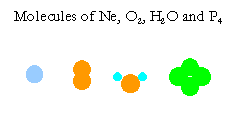# Amounts of Substances

Key Concepts

• Atoms, atomic weights, masses
• Molecules, molecular weights
• Empirical formula, ionic compounds
• Avogadro's number, mole, molar masses

Skills to Develop

• Express amounts of substances in g, kg, or mL, or L.
• Express amounts of substances in moles (mol).
• Convert amounts of substances from mass units (g, kg etc) to the unit mole.

## Amounts of Substances in Various Units

Amounts of substances are measured in units of mass (g or kg), volume (L) and mole (mol). Unit interconversions are based on the definitions of the units, and converting amounts from g or kg into mol is based on atomic masses of the elements.

Atomic masses are the masses of one mole of elements. A mole of any element has an Avogadro's number of atoms (= 6.02x1023 atoms per mole).

The natural units of substances are molecules, which are groups of atoms bonded together, except monatomic molecules of inert gases, $$\ce{He}$$, $$\ce{Ne}$$, $$\ce{Ar}$$, $$\ce{Kr}$$, $$\ce{Xe}$$, and $$\ce{Rn}$$. For example, molecules of oxygen, water, and phosphorous are $$\ce{O2}$$, $$\ce{H2O}$$, and $$\ce{P4}$$ respectively. These molecules have 2, 3, and 4 atoms respectively. Masses of one mole of substances are called molecular weights. Atomic and molecular weights are called molar masses.The above illustrates only a very small number of examples. There are millions of compounds in the world. Please think of some other compounds you know of, and write down their formulas. Then figure out the number of atoms in each mole of your compounds.

Different substances have different molecular masses. Thus, equal masses have different numbers of atoms, molecules, or moles. On the other hand, equal numbers of moles of different substances have different masses. The stoichiometric relationships among reactants and products may be complicated in units of g, but much simpler relationships are seen if we deal with units of moles or natural units of atoms and molecules.

Moles (mol) represent amounts of substances in the unit of Avogadro's number (6.022x1023) of atoms and molecules. Since empirical formulas such as $$\ce{Fe^2+}$$ ions and $$\ce{Fe2O3}$$ are used for ionic compounds, a mole represent Avogadro's number of ions or per formula as written. A mole of $$\ce{Fe^2+}$$ has 6.022x1023 ions, and a mole of $$\ce{Fe2O3}$$ has 1.204x1024 $$\ce{Fe}$$ and 1.8066x1024 $$\ce{O}$$ atoms, a total of 3.0x1024 $$\ce{Fe}$$ and $$\ce{O}$$ atoms.

The mole unit is very important for chemical reactions, as is the skill to convert masses in g to mol. The number of moles of a substance in a sample is the mass in g divided by the molar mass, which gives the amount in moles.

$$\mathrm{mole = \dfrac{mass\:(g)}{molar\: mass\: (g / mol) }}$$

Another common measure of substances is volume. Since density is the mass divided by its volume, conversion between volume and mass is accomplished by the formula:

$$\mathrm{density = \dfrac{mass\:(g)}{volume\: (cm^3)}}$$

$$\mathrm{mass = density\: (g\: cm^{-3}) \times volume\: (cm^3)}$$

These fundamental formulas are results of the definition of these terms.

## Skill Developing Questions

1. What are the molar masses of the elements hydrogen ($$\ce{H}$$), oxygen ($$\ce{O}$$), iron ($$\ce{Fe}$$) and gold ($$\ce{Au}$$)?

Know where to find: 1.0, 16.0, 55.9, 197

Skill: know where to find molar masses of elements.

2. The element gold is a precious metal. How many moles of gold are present in a mass of 1.0 kg?

1000/197 = ?

Skill: to convert mass in g into moles.

1. A liter of water has a mass of 1.0 kg. How many moles of water are 1.0 kg?

1000 g / 18 g = 55.6 mol

Skill: calculate molar masses of molecular compounds

1. At standard temperature and pressure, a mole of gas occupies 22.4 L. If 20% of air is oxygen, how many moles of oxygen are contained in 1.0 L?

0.20 mol / 22.4 L = 8.93e-3 mol/L

Skill: express amount in volume.

1. How many grams are there in 8.93e-3 mol of oxygen $$\ce{O2}$$ (molar mass 32.0)?

32 g/mol * 8.93e-3 mol = 0.286 g

Skill: convert amounts in moles to mass in g or kg.

1. How many moles of $$\ce{Fe2O3}$$ are present in 1000 kg of the oxide? Atomic weights: $$\ce{Fe}$$, 55.8, $$\ce{O}$$, 16.0.

1000000 g/(159.6 g/mol) = 6265 mol

Skill: convert amounts between moles and kg of compounds represented by chemical formulas.